Complex transient dynamics of hidden attractors in a simple 4D system*
Dang Xiao-Yua), Li Chun-Biaob), Bao Bo-Chengc)†, Wu Hua-Gand)
College of Electronic and Information Engineering, Nanjing University of Aeronautics and Astronautics, Nanjing 210016, China
School of Electronic & Information Engineering, Nanjing University of Information Science & Technology, Nanjing 210044, China
School of Information Science and Engineering, Changzhou University, Changzhou 213164, China
School of Electronic and Optical Engineering, Nanjing University of Science and Technology, Nanjing 210094, China

Corresponding author. E-mail: mervinbao@126.com

*Project supported by the National Natural Science Foundation of China (Grant No. 51277017), the Natural Science Foundation of Jiangsu Province, China (Grant No. BK2012583), and the Fundamental Research Funds for the Central Universities of China (Grant No. NS2014038).

Abstract

A simple four-dimensional system with only one control parameter is proposed in this paper. The novel system has a line or no equilibrium for the global control parameter and exhibits complex transient transition behaviors of hyperchaotic attractors, periodic orbits, and unstable sinks. Especially, for the nonzero-valued control parameter, there exists no equilibrium in the proposed system, leading to the formation of various hidden attractors with complex transient dynamics. The research results indicate that the dynamics of the system shows weak chaotic robustness and depends greatly on the initial states.

Keyword: 05.45.−a; 05.45.Ac; 05.45.Pq; transient dynamics; hidden attractor; equilibrium
1. Introduction

Due to existing hidden attractors, some particular dynamical systems related to line equilibrium or without equilibrium or stable equilibrium have received much attention in recent years. For the classical chaotic systems including the Lorenz system,  Chua’ s circuit,  and many other widely-known systems,  their attractors, called self-excited attractors, emerge from the corresponding unstable equilibria with index 2, i.e., an attractor with a basin of attraction is associated with an unstable equilibrium. However, for these particular chaotic systems, their attractors are hidden because the generations have nothing to do with any unstable equilibrium and there is no way to choose an initial condition that guarantees that they will be found.[5, 6] A hidden attractor with a small basin of attraction is not associated with an unstable equilibrium, [1, 4] which is of considerable importance in engineering applications because of the occurrence of unexpected behaviors in some real nonlinear dynamical systems,  such as Chua’ s electrical circuit, [16, 17] the drilling system actuated by an induction motor with a wound rotor,  the aircraft flight control system with input saturation,  and so on.

Like the self-excited attractors, the hidden attractors have many similar dynamical behaviors, including chaos, bifurcation, periodic window, coexisting attractors, etc.[1, 16] Some complex transient transition behaviors of the hidden attractors are rarely reported in the literature. In this paper, a simple four-dimensional (4D) system with seven terms, two quadratic nonlinearities, and one control parameter are considered, which is based on an extension of the three-dimensional (3D) robust chaotic system in Ref.  . The proposed system has a line equilibrium for the zero-valued control parameter or no equilibrium for the nonzero-valued control parameter. In fact, the triggering dynamics closely depends on the initial states for the system with a line equilibrium[4, 15] and the generating attractors are all hidden for the system without any equilibrium.[1, 3, 79] Thus, based on the above two cases, the dynamical behaviors of the proposed system will be investigated in detail in this paper.

2. New chaotic system with line equilibrium or without equilibrium

By simplifying a three-dimensional robust chaotic system in Ref.   and using a linear feedback controller, a simple 4D system is proposed and expressed aswhere μ is a unique control parameter.

The system is dissipative. The dissipativity can be derived from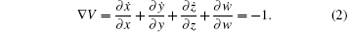Thus, system (1) is dissipative with solutions as time goes to infinity that contract onto an attractor of zero measure in 4D state space.

By setting the left-hand side to zero, we haveIt is found that there are two cases for the solutions of Eq. (3).

Case 1 When μ = 0, system (1) has an equilibrium set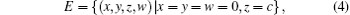where c is uncertain but constant, corresponding to the z axis. Thus, the equilibrium set E is also known as a line equilibrium.[4, 15]

Case 2 When μ ≠ 0, equation (3) has no real solutions and system (1) has no equilibrium, implying that when μ is nonzero, the generating attractors of the system are all hidden with the basin of attraction not intersecting with the small neighborhood of any equilibrium.[1, 3, 79] Furthermore, the terms on the left-hand side of Eq. (3) must be zero after the time average so that the attractors can be bounded.

For Case 1, the Jacobian matrix at the equilibrium set E is obtained asThe characteristic polynomial is derived as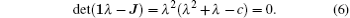Solving Eq. (6) yields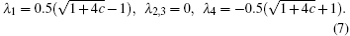It is found that when c > 0 and λ 1 > 0, i.e., the equilibrium set E is an unstable saddle point, otherwise the equilibrium set E is a stable saddle point or a stable saddle focus. The resulting eigenvalues show that the stability of the equilibrium set E has a close relationship with the initial states, leading to the dependent dynamics of system (1) associated with the initial states.[4, 15]

3. Transient hyperchaotic attractors and unstable sinks

Firstly, we consider Case 1 of μ = 0 or system (1) with a line equilibrium. The parameter z(0) in the initial states (0, 0.000001, z(0), 0) is taken as a varying parameter. With the variation of z(0) = c, the Lyapunov exponent spectra at t = 2 ks and the corresponding bifurcation diagram in the time interval [200, 400] s are depicted in Figs. 1(a) and 1(b), respectively. Here, the four Lyapunov exponents are denoted by LEi (i = 1, 2, 3, 4), with LE1 > LE2 > LE3 > LE4. For clarity, the largest three Lyapunov exponents LE1, LE2, and LE3 are presented, whereas the fourth Lyapunov exponent LE4 is omitted because its value with a large negative is independent of the system dynamics. As the initial state z(0) = c gradually increases, system (1) starts from stable sinks with the zero largest Lyapunov exponent, and then enters the complex dynamics of transient hyperchaotic attractors or steady chaotic attractors, and unstable sinks appear alternately. Thus, the system (1) is interestingly multistable and can exhibit complicated nonlinear phenomena, leading to extreme dependence of the devolvement of phase trajectories on the initial states.

 Figure Option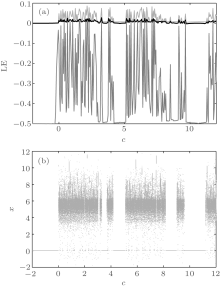Fig. 1. Dynamics with the variation of z(0) = c: (a) the largest three Lyapunov exponents; (b) bifurcation diagram of the state variable x.

The above two examples illustrate that the hyperchaotic attractors in system (1) are transient. When c = 0, the equilibrium set E of system (1) is only an origin and the corresponding four eigenvalues are λ 1 = 0, λ 2, 3 = 0, and λ 4 = − 1, implying that the origin is a critical point with uncertain stability. The phase portrait in the xy plane, the time-domain waveform of the variable z, and the largest three Lyapunov exponents for c = 0 are shown in Figs. 2(a), 2(b), and 2(c), respectively. Figure 2(a) displays the phase portrait in a limited time interval. Figure 2(b) illustrates that the dynamics of system (1) has a transition from the origin to transient hyperchaotic behaviors, and to unstable sinks. Figure 2(c) exhibits that there are two positive Lyapunov exponents LE1 and LE2 in a limited time interval and the largest Lyapunov exponent tends to be zero with time evolution, resulting in the transient hyperchaos in system (1).

 Figure Option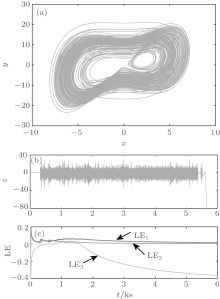Fig. 2. Transient hyperchaotic attractors for c = 0. (a) Phase portrait in the time interval [2.2, 2.5] ks. (b) Time-domain waveform of the state variable z. (c) The largest three Lyapunov exponents versus time.

When c = 6, the equilibrium set E of system (1) is a nonzero equilibrium and the four eigenvalues are calculated by Eq. (7) as λ 1 = 2, λ 2, 3 = 0, and λ 4 = − 3, which means that the nonzero equilibrium is an unstable saddle. Correspondingly, the four Lyapunov exponents are (0.0566, 0.0176, 0.0091, − 1.0834) at t = 2 ks and (0.0181, 0.0039, − 0.3609, − 0.6621) at t = 10 ks. These results show that the system (1) is transient hyperchaotic and has a transition from transient hyperchaotic to chaotic behaviors with the long time evolution. More examples of complex transient dynamical behaviors can be demonstrated by numerical simulation under different initial states of the state variable z.

Specially, the sinks with the largest zero Lyapunov exponent in the region of c < − 0.02 are stable, implying that all trajectories of the state variable z are convergent to a stable point with the time evolution; whereas the sinks with a positive largest Lyapunov exponent and a zero Lyapunov exponent in the region of c > 0 are unstable, where with the time evolution, three trajectories of the state variables x, y, and w are convergent to a stable point and the trajectory of the state variable z tends to be infinite. An example for the unstable sink is plotted in Fig. 3, where the initial state c = 10 and the four Lyapunov exponents are (0.0059, − 0.001, − 0.4945, − 0.5103) at t = 2 ks.

 Figure Option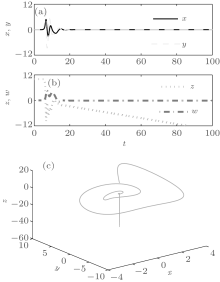Fig. 3. Unstable sinks, where the trajectory of z tends to be infinite with the time evolution: (a), (b) time-domain waveforms of four state variables; (c) corresponding trajectory in the x– y– z space.

4. Hidden attractors with complex transient behaviors

Now we consider Case 2 of μ ≠ 0 or system (1) without equilibrium. The initial states of system (1) are selected as (1, 1, 1, 1) and the control parameter μ is regarded as a varying parameter. The largest three Lyapunov exponents at t = 2 ks and the corresponding bifurcation diagram in the time interval [200, 400] s are displayed in Figs. 4(a) and 4(b), respectively. When μ gradually increases, system (1) starts from transient hyperchaotic behaviors and then degrades into chaotic behaviors, and finally settles down periodic behaviors at μ = 5.7. Two typical phase portraits within the transient hyperchaotic region and the periodic region are shown in Figs. 5(a) and 5(b), respectively. Note that these phase portraits are hidden attractors.

It is remarkable that the dynamics of system (1) without equilibrium is closely related to the initial states for different control parameters also, which results in that complex transient transition behaviors of hidden attractors occur in system (1). Two examples are illustrated in Figs. 6 and 7, respectively.

 Figure Option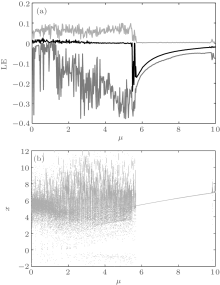Fig. 4. Dynamics of the variation of the control parameter μ : (a) largest three Lyapunov exponents; (b) bifurcation diagram of the state variable x.

 Figure Option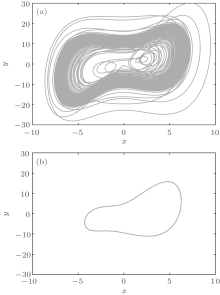Fig. 5. Projections on the x– y plane for initial states (1, 1, 1, 1). (a) Transient hyperchaotic attractor at μ = 0.52 with LEs (0.0688, 0.0177, − 0.0015, − 1.0851). (b) Limit cycle at μ = 8 with LEs (0, − 0.0388, − 0.0575, − 0.9062).

 Figure Option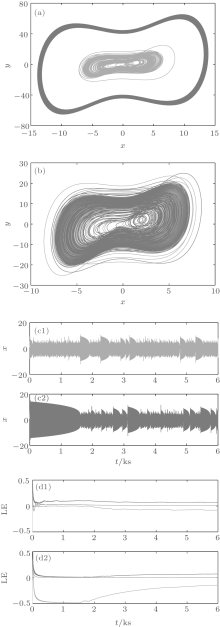Fig. 6. Hidden attractors with complex transient behaviors. (a) Projections on the x– y plane in the time interval [100 s, 400 s]. (b) Projections on the x– y plane in the time interval [3.7 ks, 4 ks]. (c) Time-domain waveform of the variable x with different initial states. (d) The largest three Lyapunov exponents against time with different initial states.

When μ = 1.5, the time-domain waveforms, the phase portraits in two different time intervals, and the largest three Lyapunov exponents against the times of two hidden attractors with different initial states are demonstrated in Figs. 6(a), 6(b), 6(c), and 6(d), respectively. In Figs. 6(a), 6(b), and 6(c), the trajectories colored in red start from the initial states (1, 1, 1, 1) and those colored in blue start from the initial states (1, 1, − 1, 1); while figures 6(d1) and 6(d2) correspond to the initial states of (1, 1, 1, 1) and (1, 1, − 1, 1), respectively.

From Fig. 6, it can be seen that when different initial states are utilized, system (1) has complicated transient transition dynamics. When the initial states are taken as (1, 1, 1, 1), the orbits of system (1) have a transition from transient hyperchaotic to steady chaotic behaviors with the time evolutions; while when the initial states are chosen as (1, 1, − 1, 1), the orbits of system (1) have a transition from transient periodic to steady chaotic behaviors with the time evolutions. These complicated transient transition behaviors can be reflected from the Lyapunov exponents against time in Fig. 6(d), where the largest two Lyapunov exponents are greater than zero for the initial states (1, 1, 1, 1) and the largest Lyapunov exponent is close to zero for the initial states (1, 1, − 1, 1) during the occurrences of transient transition behaviors, and their four different Lyapunov exponents finally converge to four identical values, respectively.

 Figure Option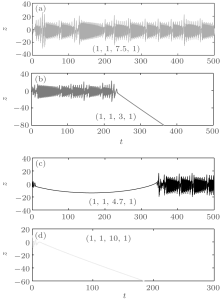Fig. 7. Time-domain waveforms of coexisting hidden attractors with different initial states. (a) Steady hyperchaotic behavior. (b) Transient hyperchaotic behavior. (c) Chaotic behavior having long transition time. (d) Unstable sinks.

Figure 7 displays the time-domain waveforms of the state variable z of the coexisting hidden attractors at μ = 0.01. In Fig. 7(a), the initial states and the Lyapunov exponents of system (1) are (1, 1, 7.5, 1) and (0.0617, 0.0204, 0, − 1.0826), respectively; in Fig. 7(b), the initial states and the Lyapunov exponents correspond to (1, 1, 3, 1) and (0.0504, 0.0174, 0, − 1.0679), respectively; in Fig. 7(c), the initial states and the Lyapunov exponents are equal to (1, 1, 4.7, 1) and (0.0158, 0, − 0.4246, − 0.5876), respectively; whereas in Fig. 7(d), the initial states and the Lyapunov exponents are equal to (1, 1, 10, 1) and (0.0042, − 0.0053, − 0.4967, − 0.5022), respectively. Observed from Fig. 7, it can be found that the complex transient transition behaviors of the coexisting hidden attractors exist in the proposed system. Note that the above four Lyapunov exponents are the values at t = 2 ks.

5. Conclusions

In conclusion, we have studied a simple four-dimensional system with seven terms, two quadratic nonlinearities, and one control parameter. The system has a line equilibrium or no equilibrium resting in the value of the control parameter and exhibits transient hyperchaos, unstable sinks, and hidden attractors over a large parameter region. Especially, the coexisting hidden attractors with complex transient transition behaviors of hyperchaotic attractors, periodic orbits and unstable sinks can be found in the proposed system. The unexpected transient transition behavior is one of the special nonlinear dynamical phenomena which should be avoided in engineering applications.

Reference
 1 Li C B and Sprott J C 2014 Int. J. Bifurc. Chaos 24 1450034 DOI:10.1142/S0218127414500345 [Cited within:6] [JCR: 0.921] 2 Zhao H T, Lin Y P and Dai Y X 2014 Int. J. Bifurc. Chaos 24 1450080 DOI:10.1142/S0218127414500801 [Cited within:1] [JCR: 0.921] 3 Pham V T, Rahma F, Frasca M and Fortuna L 2014 Int. J. Bifurc. Chaos 24 1450087 DOI:10.1142/S0218127414500874 [Cited within:2] [JCR: 0.921] 4 Jafari S and Sprott J C 2013 Chaos Soliton. Fract. 57 79 DOI:10.1016/j.chaos.2013.08.018 [Cited within:4] [JCR: 1.246] 5 Leonov G A, Kuznetsov N V and Vagaitsev V I 2011 Phys. Lett. A 375 2230 DOI:10.1016/j.physleta.2011.04.037 [Cited within:1] [JCR: 1.11] 6 Leonov G A, Kuznetsov N V and Vagaitsev V I 2012 Physica D 241 1482 DOI:10.1016/j.physd.2012.05.016 [Cited within:1] [JCR: 1.669] 7 Wei Z and Yang Q 2012 Nonlinear Dyn. 68 543 DOI:10.1007/s11071-011-0235-8 [Cited within:2] 8 Wei Z 2011 Phys. Lett. A 376 102 DOI:10.1016/j.physleta.2011.10.040 [Cited within:1] [JCR: 1.11] 9 Wang Z, Cang S, Ochola E O and Sun Y 2012 Nonlinear Dyn. 69 531 DOI:10.1007/s11071-011-0284-z [Cited within:3] 10 Stewart I 2000 Nature 406 948 DOI:10.1038/35023206 [Cited within:1] [JCR: 38.597] 11 Matsumoto T 1984 IEEE Trans. Circuits Syst. CAS-31 1055 DOI:10.1109/TCS.1984.1085459 [Cited within:1] 12 Lü J H and Chen G R 2002 Int. J. Bifurc. Chaos 12 659 DOI:10.1142/S0218127402004620 [Cited within:1] [JCR: 0.921] 13 Bao B C, Li C B, Xu J P and Liu Z 2008 Chin. Phys. B 17 4022 DOI:10.1088/1674-1056/17/11/014 [Cited within:2] [JCR: 1.148] [CJCR: 1.2429] 14 Xue W, Qi G Y, Mu J J, Jia H Y and Guo Y L 2013 Chin. Phys. B 22 080504 DOI:10.1088/1674-1056/22/8/080504 [Cited within:1] [JCR: 1.148] [CJCR: 1.2429] 15 Bao B C, Xu J P and Liu Z 2010 Chin. Phys. Lett. 27 070504 DOI:10.1088/0256-307X/27/7/070504 [Cited within:5] [JCR: 0.811] [CJCR: 0.4541] 16 Li Q D, Zeng H Z and Yang X S 2014 Nonlinear Dyn. 77 255 DOI:10.1007/s11071-014-1290-8 [Cited within:3] 17 Leonov G A and Kuznetsov N V 2013 Int. J. Bifurc. Chaos 23 1330002 DOI:10.1142/S0218127413300024 [Cited within:1] [JCR: 0.921] 18 Leonov G A, Kuznetsov N V, Kiseleva M A, Solovyeva E P and Zaretskiy A M 2014 Nonlinear Dyn. 77 277 DOI:10.1007/s11071-014-1292-6 [Cited within:1] 19 Andrievsky B R, Kuznetsov N V, Leonov G A and Pogromsky A Y 2013 Proc. IFAC 2013 75 DOI:10.3182/20130902-5-DE-2040.00040 [Cited within:2] 20 Li C B and Sprott J C 2014 Int. J. Bifurc. Chaos 24 1450131 DOI:10.1142/S0218127414501314 [Cited within:1] [JCR: 0.921]